﻿ 一类自扩散–交叉扩散离散捕食系统的斑图形成

# 一类自扩散–交叉扩散离散捕食系统的斑图形成The Pattern Formation of a Certain Kind of the Self- and Cross-Diffusion Discrete Predator-Prey System

Abstract: The spatiotemporal dynamics of Holling-II spatiotemporal discrete-time reactive self-diffusion and cross-diffusion predator-prey system was studied by constructing a three-chain coupled map lattice model. After analyzing and determining the homogeneous stable state of the spatio-temporal discrete predator-prey system, the Turing instability was analyzed and the Turing instability conditions were calculated. The numerical simulation on the basis of Turing instability showed the self-organizing process of spatial density distribution patterns, and it was found that the spatial density patterns of predator and prey always evolved into block, disk and spiral wave patterns from random distributions.

1. 引言

2. 三链CML的捕食模型构建

$\frac{\text{d}U}{\text{d}T}=\beta U\left(1-\frac{U}{K}\right)-\frac{EMUV}{A+U}$ (1a)

$\frac{\text{d}V}{\text{d}T}=V\left(\frac{MU}{A+U}-D\right)$ (1b)

$\frac{\text{d}u}{\text{d}t}=u\left(1-\frac{u}{k}\right)-\frac{uv}{a+u}$ (2a)

$\frac{\text{d}v}{\text{d}t}=v\left(\frac{bu}{a+u}-c\right)$ (2b)

$\frac{\partial u}{\partial t}-\Delta \left({d}_{1}u+{\rho }_{12}uv\right)=u\left(1-\frac{u}{k}\right)-\frac{uv}{a+u}$ (3a)

$\frac{\partial v}{\partial t}-\Delta \left({d}_{2}v+{\rho }_{21}uv\right)=v\left(\frac{bu}{a+u}-c\right)$ (3b)

$\frac{\partial u}{\partial t}=\frac{\partial v}{\partial t}=0$ (3c)

$u\left(x,0\right)={u}_{0}\left(x\right)$$v\left(x,0\right)={v}_{0}\left(x\right)$ (3d)

${{u}^{\prime }}_{\left(i,j,t\right)}={u}_{\left(i,j,t\right)}+\frac{\tau }{{h}^{2}}{d}_{1}{\nabla }_{d}^{2}{u}_{\left(i,j,t\right)}$ (4a)

${{v}^{\prime }}_{\left(i,j,t\right)}={v}_{\left(i,j,t\right)}+\frac{\tau }{{h}^{2}}{d}_{2}{\nabla }_{d}^{2}{v}_{\left(i,j,t\right)}$ (4b)

τ表示时间步长，h 表示空间步长， ${\nabla }_{d}^{2}$ 为离散拉普拉斯算子，且

${\nabla }_{d}^{2}{x}_{\left(i,j,t\right)}={x}_{\left(i+1,j,t\right)}+{x}_{\left(i-1,j,t\right)}+{x}_{\left(i,j+1,t\right)}+{x}_{\left(i,j-1,t\right)}-4{x}_{\left(i,j,t\right)}$ (5)

${{u}^{″}}_{\left(i,j,t\right)}={{u}^{\prime }}_{\left(i,j,t\right)}+\frac{\tau }{{h}^{2}}{\rho }_{12}{\nabla }_{d}^{2}{{w}^{\prime }}_{\left(i,j,t\right)}$ (6a)

${{v}^{″}}_{\left(i,j,t\right)}={{v}^{\prime }}_{\left(i,j,t\right)}+\frac{\tau }{{h}^{2}}{\rho }_{21}{\nabla }_{d}^{2}{{w}^{\prime }}_{\left(i,j,t\right)}$ (6b)

${u}_{\left(i,j,t+1\right)}={{u}^{″}}_{\left(i,j,t\right)}+\tau {{u}^{″}}_{\left(i,j,t\right)}\left(1-\frac{{{u}^{″}}_{\left(i,j,t\right)}}{k}\right)-\frac{\tau {{u}^{″}}_{\left(i,j,t\right)}{{v}^{″}}_{\left(i,j,t\right)}}{a+{{u}^{″}}_{\left(i,j,t\right)}}$ (7a)

${v}_{\left(i,j,t+1\right)}={{v}^{″}}_{\left(i,j,t\right)}+\tau {{v}^{″}}_{\left(i,j,t\right)}\left(\frac{b{{u}^{″}}_{\left(i,j,t\right)}}{a+{{u}^{″}}_{\left(i,j,t\right)}}-c\right)$ (7b)

3. 捕食系统的斑图形成条件

3.1. 不动点和稳定性

${\nabla }_{d}^{2}{u}_{\left(i,j,t\right)}\equiv 0$${\nabla }_{d}^{2}{v}_{\left(i,j,t\right)}\equiv 0$${\nabla }_{d}^{2}{w}_{\left(i,j,t\right)}\equiv 0$ (8)

$\left(\begin{array}{c}u\\ v\end{array}\right)=\left(\begin{array}{c}u+\tau u\left(1-\frac{u}{k}\right)-\frac{\tau uv}{a+u}\\ v+\tau v\left(\frac{bu}{a+u}-c\right)\end{array}\right)$ (9)

$J\left(u,v\right)=\left(\begin{array}{cc}1+\tau \left(1-\frac{2u}{k}-\frac{av}{{\left(a+u\right)}^{2}}\right)& -\frac{\tau u}{a+u}\\ \frac{\tau abv}{{\left(a+u\right)}^{2}}& 1+\tau \left(\frac{bu}{a+u}-c\right)\end{array}\right)$ (10)

$J\left({u}_{1},{v}_{1}\right)=\left(\begin{array}{cc}1-\tau & -\frac{\tau k}{a+k}\\ 0& 1+\tau \left(\frac{bk}{a+k}-c\right)\end{array}\right)$ (11)

$J\left({u}_{2},{v}_{2}\right)=\left(\begin{array}{cc}1+\frac{\tau }{k}\left(k-2{u}^{*}-\frac{a\left(k-{u}^{*}\right)}{a+{u}^{*}}\right)& -\frac{\tau {u}^{*}}{a+{u}^{*}}\\ \frac{\tau ab\left(k-{u}^{*}\right)}{k\left(a+{u}^{*}\right)}& 1+\tau \left(\frac{b{u}^{*}}{a+{u}^{*}}-c\right)\end{array}\right)$ (12)

$q=\mathrm{det}\left(J\left({u}_{2},{v}_{2}\right)\right)$。通过计算 $|{\lambda }_{1,2}|<1$ 可得到不动点 $\left({u}_{2},{v}_{2}\right)$ 稳定的条件为 $-1-q$q<1$

3.2. 图灵失稳条件

${u}_{\left(i,j,t\right)}={u}_{2}+{\stackrel{˜}{u}}_{\left(i,j,t\right)}$${v}_{\left(i,j,t\right)}={v}_{2}+{\stackrel{˜}{v}}_{\left(i,j,t\right)}$ (13)

${\stackrel{˜}{u}}_{\left(i,j,t\right)}\ll {u}_{2}$${\stackrel{˜}{v}}_{\left(i,j,t\right)}\ll {v}_{2}$ 时，将式(13)直接代入CML方程，得

$\left(\begin{array}{c}{\stackrel{˜}{u}}_{\left(i,j,t+1\right)}\\ {\stackrel{˜}{v}}_{\left(i,j,t+1\right)}\end{array}\right)=J\left({u}_{2},{v}_{2}\right)\left(\begin{array}{c}{\stackrel{˜}{u}}_{\left(i,j,t\right)}+\frac{\tau }{{h}^{2}}{d}_{1}{\nabla }_{d}^{2}{\stackrel{˜}{u}}_{\left(i,j,t\right)}+\frac{\tau }{{h}^{2}}{\rho }_{12}\left({c}_{1}{\nabla }_{d}^{2}{\stackrel{˜}{u}}_{\left(i,j,t\right)}+{c}_{2}{\nabla }_{d}^{2}{\stackrel{˜}{v}}_{\left(i,j,t\right)}\right)+\frac{{\tau }^{2}}{{h}^{4}}{\rho }_{12}\left({c}_{1}{d}_{1}{\nabla }_{d}^{2}{\nabla }_{d}^{2}{\stackrel{˜}{u}}_{\left(i,j,t\right)}+{c}_{2}{d}_{2}{\nabla }_{d}^{2}{\nabla }_{d}^{2}{\stackrel{˜}{v}}_{\left(i,j,t\right)}\right)\\ {\stackrel{˜}{v}}_{\left(i,j,t\right)}+\frac{\tau }{{h}^{2}}{d}_{2}{\nabla }_{d}^{2}{\stackrel{˜}{v}}_{\left(i,j,t\right)}+\frac{\tau }{{h}^{2}}{\rho }_{21}\left({c}_{1}{\nabla }_{d}^{2}{\stackrel{˜}{u}}_{\left(i,j,t\right)}+{c}_{2}{\nabla }_{d}^{2}{\stackrel{˜}{v}}_{\left(i,j,t\right)}\right)+\frac{{\tau }^{2}}{{h}^{4}}{\rho }_{21}\left({c}_{1}{d}_{1}{\nabla }_{d}^{2}{\nabla }_{d}^{2}{\stackrel{˜}{u}}_{\left(i,j,t\right)}+{c}_{2}{d}_{2}{\nabla }_{d}^{2}{\nabla }_{d}^{2}{\stackrel{˜}{v}}_{\left(i,j,t\right)}\right)\end{array}\right)$ (14)

${\lambda }_{1i\stackrel{¯}{j}}=4\left({\mathrm{sin}}^{2}\frac{\left(\stackrel{¯}{i}-1\right)\pi }{n}+{\mathrm{sin}}^{2}\frac{\left(\stackrel{¯}{j}-1\right)\pi }{n}\right)$ (15a)

${\lambda }_{2i\stackrel{¯}{j}}=16\left({\mathrm{sin}}^{4}\frac{\left(\stackrel{¯}{i}-1\right)\pi }{n}+{\mathrm{sin}}^{2}\frac{\left(\stackrel{¯}{i}-1\right)\pi }{n}{\mathrm{sin}}^{2}\frac{\left(\stackrel{¯}{j}-1\right)\pi }{n}\right)$ (15b)

$\begin{array}{c}\sum {X}_{\stackrel{¯}{i}\stackrel{¯}{j}}^{ij}{\stackrel{˜}{u}}_{\left(i,j,t+1\right)}={a}_{11}\sum {X}_{\stackrel{¯}{i}\stackrel{¯}{j}}^{ij}{\stackrel{˜}{u}}_{\left(i,j,t\right)}+{a}_{12}\sum {X}_{\stackrel{¯}{i}\stackrel{¯}{j}}^{ij}{\stackrel{˜}{v}}_{\left(i,j,t\right)}\\ \text{\hspace{0.17em}}\text{\hspace{0.17em}}+{a}_{11}\frac{\tau }{{h}^{2}}\left({d}_{1}\sum {X}_{\stackrel{¯}{i}\stackrel{¯}{j}}^{ij}{\nabla }_{d}^{2}{\stackrel{˜}{u}}_{\left(i,j,t\right)}+{\rho }_{12}\left({c}_{1}\sum {X}_{\stackrel{¯}{i}\stackrel{¯}{j}}^{ij}{\nabla }_{d}^{2}{\stackrel{˜}{u}}_{\left(i,j,t\right)}+{c}_{2}\sum {X}_{\stackrel{¯}{i}\stackrel{¯}{j}}^{ij}{\nabla }_{d}^{2}{\stackrel{˜}{v}}_{\left(i,j,t\right)}\right)\right)\\ \text{\hspace{0.17em}}\text{\hspace{0.17em}}+{a}_{12}\frac{\tau }{{h}^{2}}\left({d}_{2}\sum {X}_{\stackrel{¯}{i}\stackrel{¯}{j}}^{ij}{\nabla }_{d}^{2}{\stackrel{˜}{v}}_{\left(i,j,t\right)}+{\rho }_{21}\left({c}_{1}\sum {X}_{\stackrel{¯}{i}\stackrel{¯}{j}}^{ij}{\nabla }_{d}^{2}{\stackrel{˜}{u}}_{\left(i,j,t\right)}+{c}_{2}\sum {X}_{\stackrel{¯}{i}\stackrel{¯}{j}}^{ij}{\nabla }_{d}^{2}{\stackrel{˜}{v}}_{\left(i,j,t\right)}\right)\right)\\ \text{\hspace{0.17em}}\text{\hspace{0.17em}}+{a}_{11}\frac{{\tau }^{2}}{{h}^{4}}{\rho }_{12}\left({c}_{1}{d}_{1}\sum {X}_{\stackrel{¯}{i}\stackrel{¯}{j}}^{ij}{\nabla }_{d}^{2}{\nabla }_{d}^{2}{\stackrel{˜}{u}}_{\left(i,j,t\right)}+{c}_{2}{d}_{2}\sum {X}_{\stackrel{¯}{i}\stackrel{¯}{j}}^{ij}{\nabla }_{d}^{2}{\nabla }_{d}^{2}{\stackrel{˜}{v}}_{\left(i,j,t\right)}\right)\\ \text{\hspace{0.17em}}\text{\hspace{0.17em}}+{a}_{12}\frac{{\tau }^{2}}{{h}^{4}}{\rho }_{21}\left({c}_{1}{d}_{1}\sum {X}_{\stackrel{¯}{i}\stackrel{¯}{j}}^{ij}{\nabla }_{d}^{2}{\nabla }_{d}^{2}{\stackrel{˜}{u}}_{\left(i,j,t\right)}+{c}_{2}{d}_{2}\sum {X}_{\stackrel{¯}{i}\stackrel{¯}{j}}^{ij}{\nabla }_{d}^{2}{\nabla }_{d}^{2}{\stackrel{˜}{v}}_{\left(i,j,t\right)}\right)\end{array}$ (16a)

$\begin{array}{c}\sum {X}_{\stackrel{¯}{i}\stackrel{¯}{j}}^{ij}{\stackrel{˜}{v}}_{\left(i,j,t+1\right)}={a}_{21}\sum {X}_{\stackrel{¯}{i}\stackrel{¯}{j}}^{ij}{\stackrel{˜}{u}}_{\left(i,j,t\right)}+{a}_{22}\sum {X}_{\stackrel{¯}{i}\stackrel{¯}{j}}^{ij}{\stackrel{˜}{v}}_{\left(i,j,t\right)}\\ \text{\hspace{0.17em}}\text{\hspace{0.17em}}+{a}_{21}\frac{\tau }{{h}^{2}}\left({d}_{1}\sum {X}_{\stackrel{¯}{i}\stackrel{¯}{j}}^{ij}{\nabla }_{d}^{2}{\stackrel{˜}{u}}_{\left(i,j,t\right)}+{\rho }_{12}\left({c}_{1}\sum {X}_{\stackrel{¯}{i}\stackrel{¯}{j}}^{ij}{\nabla }_{d}^{2}{\stackrel{˜}{u}}_{\left(i,j,t\right)}+{c}_{2}\sum {X}_{\stackrel{¯}{i}\stackrel{¯}{j}}^{ij}{\nabla }_{d}^{2}{\stackrel{˜}{v}}_{\left(i,j,t\right)}\right)\right)\\ \text{\hspace{0.17em}}\text{\hspace{0.17em}}+{a}_{22}\frac{\tau }{{h}^{2}}\left({d}_{2}\sum {X}_{\stackrel{¯}{i}\stackrel{¯}{j}}^{ij}{\nabla }_{d}^{2}{\stackrel{˜}{v}}_{\left(i,j,t\right)}+{\rho }_{21}\left({c}_{1}\sum {X}_{\stackrel{¯}{i}\stackrel{¯}{j}}^{ij}{\nabla }_{d}^{2}{\stackrel{˜}{u}}_{\left(i,j,t\right)}+{c}_{2}\sum {X}_{\stackrel{¯}{i}\stackrel{¯}{j}}^{ij}{\nabla }_{d}^{2}{\stackrel{˜}{v}}_{\left(i,j,t\right)}\right)\right)\\ \text{\hspace{0.17em}}\text{\hspace{0.17em}}+{a}_{21}\frac{{\tau }^{2}}{{h}^{4}}{\rho }_{12}\left({c}_{1}{d}_{1}\sum {X}_{\stackrel{¯}{i}\stackrel{¯}{j}}^{ij}{\nabla }_{d}^{2}{\nabla }_{d}^{2}{\stackrel{˜}{u}}_{\left(i,j,t\right)}+{c}_{2}{d}_{2}\sum {X}_{\stackrel{¯}{i}\stackrel{¯}{j}}^{ij}{\nabla }_{d}^{2}{\nabla }_{d}^{2}{\stackrel{˜}{v}}_{\left(i,j,t\right)}\right)\\ \text{\hspace{0.17em}}\text{\hspace{0.17em}}+{a}_{22}\frac{{\tau }^{2}}{{h}^{4}}{\rho }_{21}\left({c}_{1}{d}_{1}\sum {X}_{\stackrel{¯}{i}\stackrel{¯}{j}}^{ij}{\nabla }_{d}^{2}{\nabla }_{d}^{2}{\stackrel{˜}{u}}_{\left(i,j,t\right)}+{c}_{2}{d}_{2}\sum {X}_{\stackrel{¯}{i}\stackrel{¯}{j}}^{ij}{\nabla }_{d}^{2}{\nabla }_{d}^{2}{\stackrel{˜}{v}}_{\left(i,j,t\right)}\right)\end{array}$ (16b)

${\stackrel{¯}{u}}_{t}=\sum {X}_{\stackrel{¯}{i}\stackrel{¯}{j}}^{ij}{\stackrel{˜}{u}}_{\left(i,j,t\right)}$${\stackrel{¯}{v}}_{t}=\sum {X}_{\stackrel{¯}{i}\stackrel{¯}{j}}^{ij}{\stackrel{˜}{v}}_{\left(i,j,t\right)}$ (17)

$\left(\begin{array}{c}{\stackrel{¯}{u}}_{t+1}\\ {\stackrel{¯}{v}}_{t+1}\end{array}\right)=\left(\begin{array}{cc}{A}_{11}& {A}_{12}\\ {A}_{21}& {A}_{22}\end{array}\right)\left(\begin{array}{c}{\stackrel{¯}{u}}_{t}\\ {\stackrel{¯}{v}}_{t}\end{array}\right)$ (18)

${A}_{11}={a}_{11}-\frac{\tau }{{h}^{2}}{\lambda }_{1i\stackrel{¯}{j}}\left({a}_{11}{d}_{1}+{a}_{11}{\rho }_{12}{c}_{1}+{a}_{12}{\rho }_{21}{c}_{1}\right)-\frac{{\tau }^{2}}{{h}^{4}}{\lambda }_{2i\stackrel{¯}{j}}{c}_{1}{d}_{1}\left({a}_{11}{\rho }_{12}+{a}_{12}{\rho }_{21}\right)$ (19a)

${A}_{12}={a}_{12}-\frac{\tau }{{h}^{2}}{\lambda }_{1i\stackrel{¯}{j}}\left({a}_{12}{d}_{2}+{a}_{11}{\rho }_{12}{c}_{2}+{a}_{12}{\rho }_{21}{c}_{2}\right)-\frac{{\tau }^{2}}{{h}^{4}}{\lambda }_{2i\stackrel{¯}{j}}{c}_{2}{d}_{2}\left({a}_{11}{\rho }_{12}+{a}_{12}{\rho }_{21}\right)$ (19b)

${A}_{21}={a}_{21}-\frac{\tau }{{h}^{2}}{\lambda }_{1i\stackrel{¯}{j}}\left({a}_{21}{d}_{1}+{a}_{21}{\rho }_{12}{c}_{1}+{a}_{22}{\rho }_{21}{c}_{1}\right)-\frac{{\tau }^{2}}{{h}^{4}}{\lambda }_{2i\stackrel{¯}{j}}{c}_{1}{d}_{1}\left({a}_{21}{\rho }_{12}+{a}_{22}{\rho }_{21}\right)$ (19c)

${A}_{22}={a}_{22}-\frac{\tau }{{h}^{2}}{\lambda }_{1i\stackrel{¯}{j}}\left({a}_{22}{d}_{2}+{a}_{21}{\rho }_{12}{c}_{2}+{a}_{22}{\rho }_{21}{c}_{2}\right)-\frac{{\tau }^{2}}{{h}^{4}}{\lambda }_{2i\stackrel{¯}{j}}{c}_{2}{d}_{2}\left({a}_{21}{\rho }_{12}+{a}_{22}{\rho }_{21}\right)$ (19d)

${\stackrel{¯}{\lambda }}_{±}=\frac{1}{2}\left(\left({A}_{11}+{A}_{22}\right)±\sqrt{{\left({A}_{11}-{A}_{22}\right)}^{2}+4{A}_{12}{A}_{21}}\right)$ (20)

$L=\underset{\stackrel{¯}{i},\stackrel{¯}{j}}{\mathrm{max}}\left({\stackrel{¯}{\lambda }}_{+},{\stackrel{¯}{\lambda }}_{-}\right)>1$ (21)

4. 数值模拟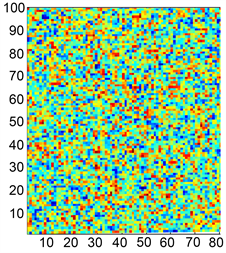(a) t = 100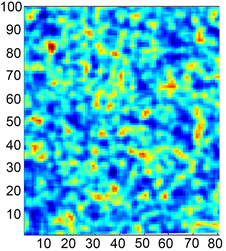(b) t = 800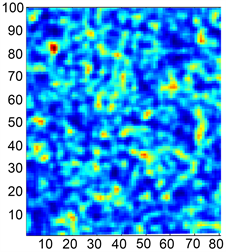(c) t = 1000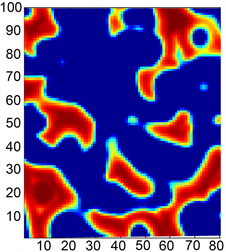(d) t = 5000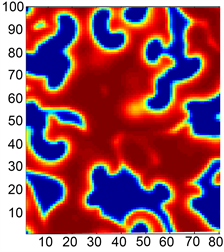(e) t = 8000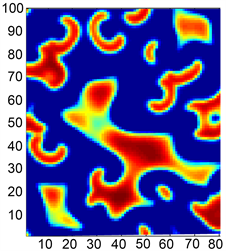(f) t = 10000

Figure 1. Pattern formation of block pattern image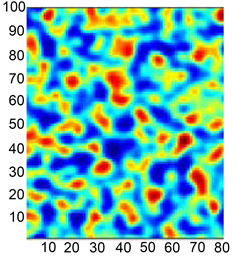(a) t = 100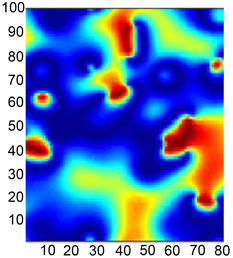(b) t = 500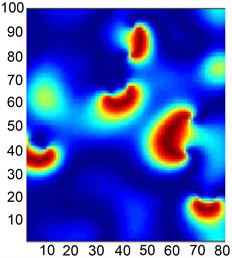(c) t = 800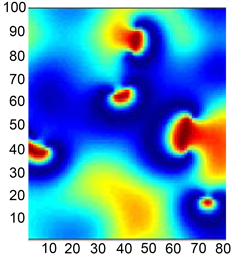(d) t = 1000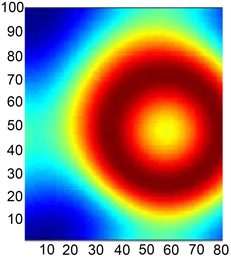(e) t = 5000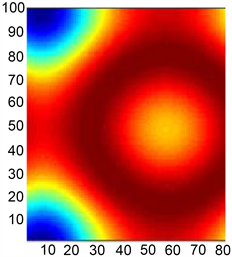(f) t = 10000

Figure 2. Pattern formation of disk pattern image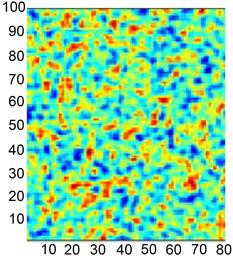(a) t = 10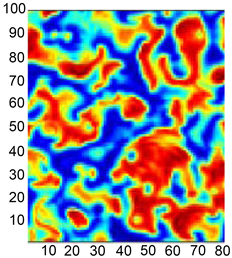(b) t = 100(c) t = 1000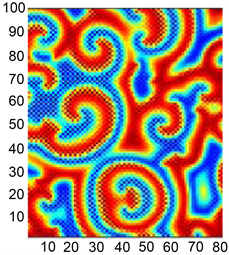(d) t = 2000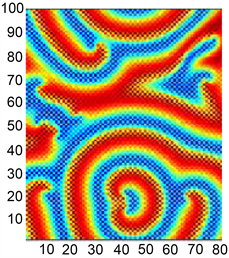(e) t = 8000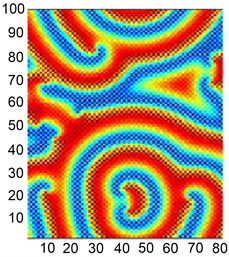(f) t = 10000

Figure 3. Pattern formation of spiral wave pattern image

NOTES

*通讯作者。

 饶凤. 随机种群动力系统研究[D]: [博士学位论文]. 上海: 华东师范大学, 2012.

 Chen, X., Fu, X. and Jing, Z. (2013) Dynamics in a Discrete-Time Predator-Prey System with Allee Effect. Acta Mathematicae Applicatae Sinica (English Series), 29, 143-164.
https://doi.org/10.1007/s10255-013-0207-5

 唐晓栋. 多反馈反应扩散系统斑图动力学研究[D]: [博士学位论文]. 徐州: 中国矿业大学, 2014.

 Sambath, M. and Balachandran, K. (2012) Pattern Formation for a Ratio-Dependent Predator-Prey Model with Cross Diffusion. Journal of the Korean Society for Industrial and Applied Mathematics, 16, 249-256.
https://doi.org/10.12941/jksiam.2012.16.4.249

 王晓丽. 带有交叉扩散项的捕食-食饵系统的研究[D]: [硕士学位论文]. 西安: 西安工程大学, 2017.

 Bie, Q., Wang, Q. and Yao, Z. (2014) Cross-Diffusion Induced Instability and Pattern Formation for a Holling Type-II Predator-Prey Model. Applied Mathematics and Computation, 247, 1-12.
https://doi.org/10.1016/j.amc.2014.08.088

 黄头生. 基于耦合映像格子的生态学时空复杂性研究[D]: [博士学位论文]. 北京: 华北电力大学, 2016.

 杨维明. 时空混沌和耦合映象格子[M]. 上海: 上海科技教育出版社, 1994.

 邹荣. 反应扩散捕食模型的动力学研究[D]: [博士学位论文]. 长沙: 湖南大学, 2018.

 Nayfeh, A.H. and Balachandran, B. (1995) Applied Nonlinear Dynamics: Analytical, Computational, and Experimental Methods. Wiley Interscience, New York, 61-67.
https://doi.org/10.1002/9783527617548

 Bai, L. and Zhang, G. (2009) Nontrivial Solutions for a Nonlinear Discrete Elliptic Equation with Periodic Boundary Conditions. Applied Mathematics and Computation, 210, 321-333.
https://doi.org/10.1016/j.amc.2008.12.024

Top